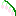# Analyze

Nodes to further ananlysis and characterization of cytometry data (eg. gating, sne, clustering)

This category contains 5 nodes.

##### Calculate StatisticsDeprecated

Calculate summary for subsets defined in an FCS data frame.

##### Extract Data

Extracts data from an FCS frame column to a standard KNIME Table. Data may be transformed and gating information included for downstream ML applications.

##### Learn TreeSNE

Calculates a tSNE using library developed by Leif Jonsson: * nhttps://github.com/lejon/T-SNE-Java

##### TSNE

Calculates a tSNE using library developed by Leif Jonsson: * nhttps://github.com/lejon/T-SNE-Java